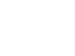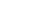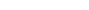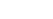## Chemistry Paper 2 Questions and Answers - Form 3 Mid Term 2 Exams 2021

Instructions to candidates.

• Answer all the questions in the spaces provided in question paper.
• Mathematical tables and silent calculators may be used.
• All working must be clearly shown where necessary.
1.
1. Study the table below which shows properties of elements across period three and answer the question that follow.
 Element A B C D E F G H Atomic radius (nm) 0.156 0.136 0.125 0.118 0.110 0.104 0.099 Ionic r Radius (nm) 0.095 0.065 0.050 0.184 0.181 Melting points (°C) 97.8 650 660 1410 44.2 119 −101 −186

1. Explain why the atomic radius of G is smaller than its ionic radius. (1mk)
2. Explain why the melting point of D is higher than the other elements (2mks)
3. Give the formula of the chlorides of element B (1mk)
2. The ions of V3+ and U2- have identical electronic configuration 2.8.
Write down the electron arrangement of the elements U and V (2mks)
U ………………………………………………………………………………………
V ………………………………………………………………………………………
3. The diagram below shows how magnesium reacts with steam1. Gas C would not be produced as is in the set-up but when certain condition is introduced gas C is produced. Identify the condition which was omitted in the set-up (1mk)
2. Describe how gas C is produced after the mistake was corrected in the above set-up (2mk)
3. Why is it not advisable to use potassium in place of magnesium? (1mk)
2. The flow chart below shows the manufacture of sodium carbonate. Study it carefully and answer the questions that follow.1.
1. What is ammonical brine? (1mk)
2. Ammonical brine reacts with carbon (IV) oxide to form a mixture of two salts which produce Q. Write an equation to show formation of Q (1mk)
3. Name two processes that are used to separate Q into NH4Cl and P(2mks)
2. Give two uses of sodium carbonate produced in the process (2mks)
3.
1. Name the substance that reacts with water that comes into the slaker (1mk)
2. What happens at the kiln? (1mk)
4. Write an equation for the reaction that occurs when P is heated to form solid
Na2CO3 (1mk)
5. Name two substances that are recycled in the process. (1mk)
6. Solvay tower is as shown below. Study the diagram and answer the question belowGive two reasons why the baffles are used in the solvay (2mks)
7. A factory produces 63.6 tonnes of anhydrous Na2CO3 on a certain day by this process.
Calculate the number of tonnes of sodium chloride used upon on this particular day.
Assume the plant is working at 100% efficiency.
(C = 12, H = 1, Cl = 35.5, Ca = 40, Na = 23) (3mks)
3. A student set-up the arrangement below to prepare and collect dry hydrogen gas1. Identify two errors from the section of the arrangement shown above(2mks)
2. Complete the diagram to show how dry hydrogen gas can be collected.(2mks)
3.
1. Explain the effect of hydrogen gas on a wet red litmus paper(1mk)
2. Write a balanced chemical equation for the reaction that takes place when hydrogen gas is burnt in air. (1mk)
4. Determine the relative atomic mass of zinc, given that when 6.54g of zinc was used, 2.4litres of hydrogen gas was produced. (molar gas volume = 24 litres) (3mks)
5. State any two non-industrial uses of hydrogen gas (1mk)
4.
1. Name the following compounds(1mk)
1.2. H2SO4
2. The diagram below is for the preparation of ethene gas in the school laboratory.1. Name substance (1mk)
X_____________________________
Y_____________________________
2. What is the use of substance Y?
3. State one condition missing in the set up (1mk)
4. Explain why ethene can’t be collected by either upward or downward delivery.(1mk)
5. Write down an equation for the production of ethene in the above set up
(1mk)
3. Study the scheme below and answer the questions that follow.1. Identify the catalyst used in step I (1mk)
2. Name the compounds A and B (1mk)
A____________________________
B_____________________________
3. Give one disadvantage of compound formed in step III (1mk)
4. Name the reactions taking place at steps: (1mk)
III______________________________
IV______________________________
5. Describe how substance K is converted to ethanol (2mks)
6. Under certain conditions, heptane can be converted to two products. The formula of one of the products is C4H10. Write down the structural formula of the other products. (1mk)
5.
1. Carbon has two allotropes. What is meant by the term allotropy? (1mk)
2. The following diagrams show the structures of two allotropes of carbon. Study them and answer the questions that follow.1. Name the allotrope (2mks)
D
E
2. Give one use of D (1mk)
3. Which allotrope does not conduct electricity. Explain (2mks)
3. State two properties of Carbon (IV) Oxide that make it suitable for use in fire extinguishers. (2mks)
4. In an experiment, Carbon (IV) Oxide gas was passed over heated coke and the gas produced collected as shown in the diagram below.1. Write an equation for the reaction that took place in the combustion tube. (1mk)
2. Name another substance that can be used instead of Potassium Hydroxide. (1mk)
3. Describe a simple chemical test that can be used to distinguish Carbon (II) oxide. (2mks)
4. Give one use of carbon(II) Oxide (1mk)
6.
1. The set up below was used to obtain dry nitrogen gas from air .study it and answer the questions that follows.1. name the method used above.(1mks)
2. identify solution q.(1mks)
3. state the observation made in the combustion tube .(1mks)
4. What is the function of concentrated sulphuric acid in the set up above.(1mks)
5. Name 1 impurity in the nitrogen gas collected by this method.(1mks)
2. The set up below was used to prepare Nitric(v)acid in the laboratory using concentrated sulphuric acid on potassium nitrate crystals.study it and answer the questions that follows.1. Explain why sodium nitrate is not appropriate option in place of potassium nitrate.(1mk)
2. A reddish brown gas was observed in the retort.explain.(1mk)
3. 60-50 % Nitric(V) acid is produced from the absorption chamber in the industrial manufacture of the nitric acid.Describe how the percentage of the acid can be increased      (2mks)
7.
1. The diagram below represents a set up used by a student to investigate the effect of heat on sodium nitrate.use it to answer the questions that follow.1. Write the chemical equation of the reaction in the boiling tube(2mks)
2. state the property of the gas that makes it to be collected by the method shown.(1mk)
3. predict the effect of water in the trough on the litmus paper after the experiment(2mks)
4. Study the set up below and answer the questions that follows.1. State and explain the observations that would be made when the circuit is completed.(3mks)
2. Name the electrodes A and B
A…………………………
B………………………………….
8.
1. 8.4g of sodium hydrogen carbonate are completely decomposed by heating.calculate the mass of the resulting solid and the volume in litres of the gas produced at s.t.p.(molar gas volume 22.4litres).
1. write a balanced chemical equation(2mks)
2. calculate the mass of solid formed(2mks)
3. calculate the volume of the gas produced in litres at s.t.p(2mks)
2. During an experiment on the reduction of an oxide of copper the following data was obtained.
Mass of empty boat…………………………………25.0g
Mass of empty boat + oxide of copper………………..29.0g
Mass of boat+copper(after reaction)……………………28.2g
(Cu=64.0 0=16)
Find the emphirical formula of copper oxide.(4mks)
3. A volume of 375cm3 of a gas has a pressure of 20 atmospheres.what will be its volume if pressure is reduced to 15 atmospheres?(3mks)## MARKING SCHEME

1.
1.
1. C gains electrons to✓½ form ions, innermost electrons✓½ repels incoming electrons hence larger ionic radius.
2. It has giant atomic✓½ structures revealed by strong covalent✓½ bonds hence require higher ✓energy to melt
3. BCl2 ✓1
2. U – 2.6✓1
V – 2.8.3 ✓1
3.
1. Heating of cotton✓½ wool and magnesium✓½
2. Wet cotton produced✓½ steam when heated ✓½
Steam reacted with hot✓½ magnesium forming gas C✓½
3. Potassium is more reactive✓1
2.
1.
1. A mixture of brine // conc NaCl and ammonia gas✓1
2. NaCl(aq) + NH3(aq) + CO2(g) + H2O(l) → NaHCO3(s) + NH4Cl(aq) ✓1
3. Filtration ✓1
Fractional crystallization ✓1
2.
• Manufacture of glass ✓1
• Cattle licks✓1
• Softening of water✓1
(Any two @ 1mk Total = 2mks)
3.
1. CaO / Calcium oxide✓1
2.
• Heating of calcium carbonate to produce CO2(g)✓1
• Heating of carbon to CO2(g)✓
(Any one)
4. 2NaHCO3(s) → Na2CO3(s) + CO2(g) + H2O(l) ✓1
5. Ammonia✓½ and carbon (IV) oxide✓½
6. Allows slow movement of ammonical brine✓1 inorder for the reaction to occur effectively// increase surface area for reaction✓1
7. NaCl(aq) + NH3(g) + CO2(g) + H2O(l) → NaHCO3(s) + NH4Cl(aq)
2NaHCO3(s) → Na2CO3(s) + CO2(g) + H2O(l) ✓1
63.6 tonnes.
Mass of Na2CO3 = 106g
Moles of Na2CO3 = 63.6 × 1000 × 1000 ✓½
106
= 600000 moles✓½
Moles of NaHCO3 = 600000 x 2
= 1200000 moles✓½
1 mol of NaCl produces 1 mol of NaHCO3
Mass of NaCl = 58.5g✓½
58.5g = 1 mol of NaHCO3
1200000 mol ✓½
= 58.5 × 1200000
1
= 70200000  g
1000×1000
=70.2tonnes ✓½
OR
Mass of NaHCO3 = 23 + 1 + 12 + 48
= 84g✓½
2 x 84g NaHCO3 = 106g Na2CO3
63.6 tonnes✓½
2 × 84 × 63.6
106
100.8 tonnes ✓ ½
Mass of NaCl = 58.5 ✓½      mass of NaHCO3 = 84✓½
58.5g NaCl = 84g NaHCO3
100.8 tonnes
58.5 × 100g
84
= 70.2✓½
3.
1. The outlet delivery tube should not dip into the Zinc/dilute Sulphuric acid mixture in the round buttoned flask. ✓1mk
2. The use of heat is not required ✓ 1mk
1.2.
1. It does not react with conc. H2SO4 ✓1 mk
2. H2(g) + ½O2(g)H2O(g)
balancing ½ mark
states ½ mark
3. Zn2(s) + H2SO4(aq) → ZnSO4(aq) + H2(g)
balancing ½ mk
states ½ mk
1vol     1 vol     1vol
6.54                  2.4
R                     24
Therefore, 6.54 = 2.4 ✓1mk where R = R.A.M of Zinc
R       24
R = 24 × 6.54
2.4
Or R = 65.4 ✓1mk
4.
• H2(g) is used in balloons by meteorologists ✓1mk
• It is used as rocket fuel ✓1mk
4.
1.
1. 2 – bromobutane✓½
2. Sulphuric (VI) acid✓
2.
1. X – Concentrated sulphuric (VI) acid✓½
Y – Concentrated sodium hydroxide / potassium hydroxide✓½
2. To absorb CO2 / SO2 formed during the reaction✓½
3. Heating ✓1
4. Its density is almost the same as that of air✓1
5.              conc H2SO4
C2H5OHC2H4(g) + H2O(l)
3.
1. Nickel✓1
2. A – Ethane ✓
B – Chloroethene✓
3.
• Produce toxic gases when burnt✓
4. III – Polymerisation✓
IV – Substitution ✓
5. By hydration of K✓ at 900°C using phosphorous (V) acid as✓ a catalyst
OR
Hydrolysis of K by adding ✓conc H2SO4 followed by water and then warmed✓
4. C3H6 ✓1
5.
1. Allotropy is the existence of an element in more than one form without a change of state
2.
1. D – Graphite (1)
E _ Diamond (1)
2. In electrolysis as an electrode or used as a lubricant Lead pencils or Atomic piles (any one) (1)
3. E or Diamond (1); all its 4 outermost electrons are involved in bonding ( ½ )
Thus it has no free/mobile electron to conduct electricity. ( ½ )
3.
• CO2(g) is denser than air (1)
• CO2(g) does not support combustion (1)
• CO2(g) does not burn (1) (any two)
4.
1. CO2(g) + C(s) → 2CO(g) (1)
2. Sodium hydroxide (1)
3. pass a sample of each gas CO2(g) and CO(g) )in a boiling tube containing Ca(OH)2(aq). Carbon(IV) Oxide (1) forms a white precipitate with Calcium Hydroxide (lime water. Carbon(II) Oxide has no reaction with Calcium Hydroxide solution (2mks)
OR
Carbon(IV) Oxide turns litmus paper pale red while Carbon(II) Oxide has no effect on litmus paper (any other correct description)
4. Reduction of metal oxides
6.
1.
1. Isolation of nitrogen from air
2. concentrated sodium hydroxide or KOH(aq)
3. Burns to produce white residue or solid
4. to remove the water vapour or to dry the gas
5. rare gases – neon and argon
2.
1. it is hygroscopic and absorbs water from air, the water reacts explosively with concentrated sulphuric acid
2. Nitric(vi) acid decomposes to form NO2 and O2 on heating the NO2 is the brown gas.
3. percentage of acid can be increased through fractional distillation.
7.
1.
1. 2NaNO3(s)NaNO2(s) + O2(g)
2. Slightly soluble in water
3. No effect on the litmus paper because the gas produced is neutral.
2.
1. when the circuit is completed the bulb lights(½) brown substance(½)formed grey(½)substance formed on cathode:because PbBr2 acts as an electrolyte(½):lead ions gain electrons to form pb(½) and loses electrons to form (Br) ½mk
2. A - cathode
B - anode
8.
1.
1. 2NaHCO3(s)  → Na2CO3(s) + CO2(g) + H2O(g)   (2mks)
2. 168 g of NaHCO3 yield 106g of Na2CO3
8.4g……………………………….106 x 8.4   =5.3g of Na2CO3(2mks)
168
3. 22.4 liters at s.t.p 168 g of NaHCO3 evolve 22.4 liters at s.t.p 8.4g of NaHCO3 evolve
22.4 x 8.4  =1.12 litres     (2mks)
168

2.  Element C O Composition 3.2 0.8 Reacting moles 3.2/64 = 0.05 0.8/16 = 0.05 Mole ratio 0.05 = 1  0.05 0.05 = 1 0.05
Emphirical formula CuO 1:1 (4mks)
3. boyles law
p1v1 =p2v2 v2 =
p1=20 atm 15
v1=375cm3
p2 = 15 atm
v2 =?
20 x 375 =15 x v2
v2 = 20 × 375
15
v2 = 500cm3  (3mks)

• ✔ To read offline at any time.
• ✔ To Print at your convenience
• ✔ Share Easily with Friends / Students